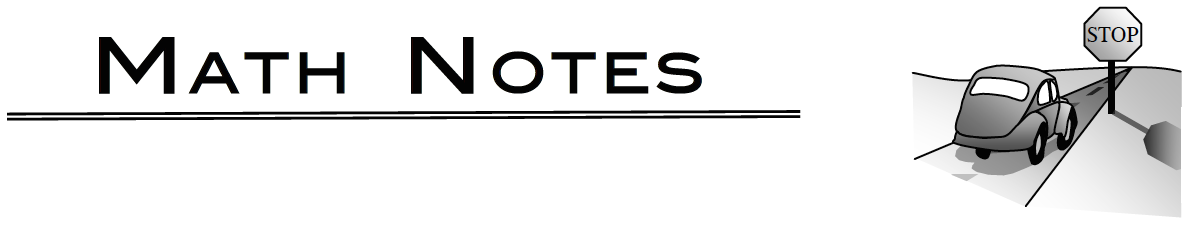### Home > PC3 > Chapter 11 > Lesson 11.2.5 > Problem11-153

11-153.

Given $z_1=$$3(\cos(\frac{\pi}{2}+i\sin(\frac{\pi}{2}))$ and$z_2=$$9(\cos(\frac{3\pi}{4}+i\sin(\frac{3\pi}{4}))$, evaluate $z_{1}z_{2}$ and $\frac { z 1 } { z _ { 2 } }$. Express your answers in both trigonometric (polar) and $a + bi$ forms.### Products and Quotients of Complex Numbers in Polar Form

Given two complex numbers $z_1 = r_1(\cos(a) + i \sin(a))$ and $z_2 = r_2(\cos(b) + i \sin(b))$.

The product of the two numbers is $z_1z_2 = r_1r_2(\cos(a + b) + i \sin(a + b))$.

The quotient of the two numbers is $\frac { z _ { 1 } } { z _ { 2 } } = \frac { r _ { 1 } } { r _ { 2 } }(\cos(a − b) + i \sin(a − b))$.